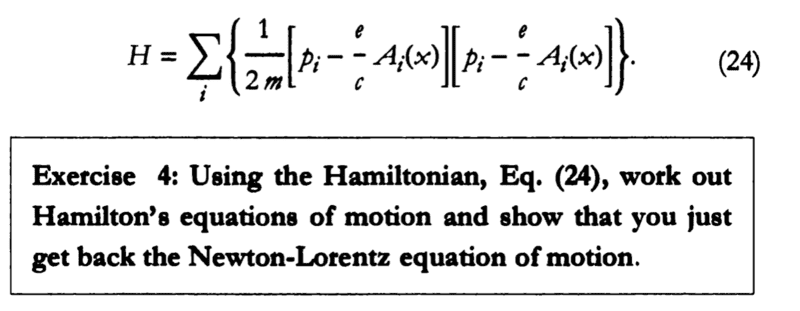# Hamiltonian for a charged particle in a magnetic field

zhangnous
I find a exercise in Leonard Susskind's book Classical Mechanicsthe Hamiltonian of a charged particle in a magnetic field(ignore the electric field) is $$H=\sum_{i} \left\{ \frac{1}{2m} \left[ p_{i}-\frac{e}{c}A_{i}(x) \right]\left[ p_{i}-\frac{e}{c}A_{i}(x) \right] \right\},$$and$$p_{i}=m\dot x_{i}+\frac{e}{c}A_{i}(x)$$and Hamilton's equations of motion is $$\dot q_{i} = \frac{\partial H}{\partial p_{i}}$$$$\dot p_{i} = -\frac{\partial H}{\partial q_{i}}$$then I solve these equations in x. $$\dot x = \frac{\partial H}{\partial p_{x}} = \frac{p_{x}-\frac{e}{c}A_{x}}{m}$$and$$\dot p_{x}=m\ddot x + \frac{e}{c}\left( \frac{\partial A_{x}}{\partial x}\dot x+\frac{\partial A_{x}}{\partial y}\dot y+\frac{\partial A_{x}}{\partial z}\dot z \right)$$$$-\frac{\partial H}{\partial x}=-\left[ \frac{p_{x}-\frac{e}{c}A_{x}}{m}(-\frac{e}{c}\frac{\partial A_{x}}{\partial x})+\frac{p_{y}-\frac{e}{c}A_{y}}{m}(-\frac{e}{c}\frac{\partial A_{y}}{\partial x})+\frac{p_{z}-\frac{e}{c}A_{z}}{m}(-\frac{e}{c}\frac{\partial A_{z}}{\partial x}) \right]=\frac{e}{c}\left( \frac{\partial A_{x}}{\partial x}\dot x+\frac{\partial A_{y}}{\partial x}\dot y+\frac{\partial A_{z}}{\partial x}\dot z \right)$$then$$m\ddot x + \frac{e}{c}\left( \frac{\partial A_{x}}{\partial x}\dot x+\frac{\partial A_{x}}{\partial y}\dot y+\frac{\partial A_{x}}{\partial z}\dot z \right)=\frac{e}{c}\left( \frac{\partial A_{x}}{\partial x}\dot x+\frac{\partial A_{y}}{\partial x}\dot y+\frac{\partial A_{z}}{\partial x}\dot z \right)$$and get $$ma_{x}=\frac{e}{c}(B_{z}\dot y-B_{y}\dot z)$$

Am I a right way to work it out? I am not sure if it is right, because it seems like a math structure game.

•vanhees71

Looks absolutely fine! The first Hamilton equation gives ##\dot{x}_i = \frac{1}{m} \left( p_i - \frac{e}{c}A_i \right)## and the second Hamilton equation gives\begin{align*}\dot{p}_i = m\ddot{x}_i + \frac{e}{c} \sum_j \left[ \frac{\partial A_i}{\partial x_j} \dot{x}_j \right] &=\frac{e}{mc} \sum_j \left[ \left( p_j - \frac{e}{c}A_j \right) \frac{\partial A_j}{\partial x_i} \right]\\

&= \frac{e}{c} \sum_j \left[ \frac{\partial A_j}{\partial x_i} \dot{x}_j \right]\end{align*}so you end up with\begin{align*}m\ddot{x}_i = \frac{e}{c} \sum_j \left[ \left( \frac{\partial A_j}{\partial x_i} - \frac{\partial A_i}{\partial x_j} \right) \dot{x}_j \right] = \frac{e}{c} \sum_j \left[ F_{ij} \dot{x}_j \right] = \frac{e}{c} \left(\mathbf{v} \times \mathbf{H} \right)_i
\end{align*}Note that in this last equation the indices of the Maxwell tensor ##F_{ij}## run over the set ##\{ 1,2,3 \}##, so here we are only dealing with magnetic components. To obtain the entire Lorentz force, you need to modify the original Hamiltonian to include an additional term ##e\phi##.

Last edited by a moderator:
•vanhees71, Twigg and zhangnous
zhangnous
Looks absolutely fine! The first Hamilton equation gives ##\dot{x}_i = \frac{1}{m} \left( p_i - \frac{e}{c}A_i \right)## and the second Hamilton equation gives\begin{align*}\dot{p}_i = m\ddot{x}_i + \frac{e}{c} \sum_j \left[ \frac{\partial A_i}{\partial x_j} \dot{x}_j \right] &=\frac{e}{mc} \sum_j \left[ \left( p_j - \frac{e}{c}A_j \right) \frac{\partial A_j}{\partial x_i} \right]\\

&= \frac{e}{c} \sum_j \left[ \frac{\partial A_j}{\partial x_i} \dot{x}_j \right]\end{align*}
So the first Hamilton equation gives ##\dot{x}_i = \frac{1}{m} \left( p_i - \frac{e}{c}A_i \right)##, which is just the canonical momentum ##p_{i}=m\dot x_{i}+\frac{e}{c}A_{i}## in this special situation, and can also be used into the second Hamilton equation. It seems strange for me and looks like these two equation play with themselves.

But I remember the situation of one free particle. Hamiltonian is ##H = \frac{p^2}{2m} + V(x)##. First gives##\dot x = \frac{\partial H}{\partial p}=\frac{p}{m}## and ##\dot p = m\ddot x## then second gives ##\dot p = -\frac{\partial H}{\partial x}=-\frac{\partial V(x)}{\partial x}=F##. Finally have Newton's equation ##F=m\ddot x##. Which is also the two equation play with each other. This seems I just playing a joke with my own mind.

Thanks for you comprehensive explanation

•vanhees71, Twigg and etotheipi
Hamilton's equations should be equivalent to Newton's laws in these cases. You can derive Lagrange's equations either through variational principles or by d'Alembert's principle. With Einstein summation convention (all following formulae assume summation over repeated indices), the Lagrangian itself satisfies ##d\mathcal{L} = \frac{\partial \mathcal{L}}{\partial q^i} dq^i + \frac{\partial \mathcal{}}{\partial \dot{q}^i} d\dot{q}^i = \dot{p}_i dq^i + p_i d\dot{q}^i##. You can do a Legendre transform ##\mathcal{H} := p_i \dot{q}^i - \mathcal{L}## from which it follows that ##d\mathcal{H} = -\dot{p}_i dq^i + \dot{q}^i dp_i## and by comparison to the expression for the total derivative of ##\mathcal{H}## you identify ##\dot{q}^i = \partial \mathcal{H} / \partial p_i## and ##\dot{p}_i = - \partial \mathcal{H} / \partial q^i##.

It all follows over to classical field theory, e.g. for a particle interacting with an electromagnetic field you have a Lagrangian $$\mathcal{L} = -mc^2 \sqrt{1-\frac{v^2}{c^2}} + \frac{e}{c} A_i v^i - e\phi$$Defining ##P^i = mv^i/\sqrt{1-\frac{v^2}{c^2}} + \frac{e}{c}A^i## one can check that this gives $$\mathcal{H} = e\phi + \sqrt{m^2c^4 + \left(P_i - \frac{e}{c} A_i \right) \left(P^i - \frac{e}{c} A^i \right) }$$which in the limit ##v/c \rightarrow 0## reduces to ##\mathcal{H} = e\phi + \frac{1}{2m} \left(P_i - \frac{e}{c} A_i \right) \left(P^i - \frac{e}{c} A^i \right)##, i.e. Susskind's expression just with the ##e\phi## term included. Also notice that ##\lim_{v/c \rightarrow 0} P^i = p^i = mv^i + \frac{e}{c}A^i##.

Last edited by a moderator:
••vanhees71, zhangnous and Twigg